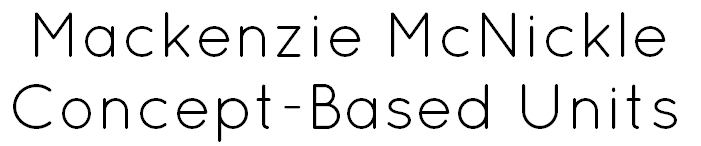Back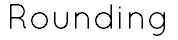MAFS.4.NBT.1.1: Recognize that in a multi-digit whole number, a digit in one place represents ten times what it represents in the place to its right. For example, recognize that 700 ÷ 70 = 10 by applying concepts of place value and division. MAFS.4.NBT.1.2: Read and write multi-digit whole numbers using base-ten numerals, number names, and expanded form. Compare two multi-digit numbers based on meanings of the digits in each place, using >, =, and < symbols to record the results of comparisons. MAFS.4.NBT.1.3: Use place value understanding to round multi-digit whole numbers to any place.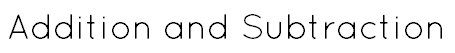MAFS.4.NBT.2.4: Fluently add and subtract multi-digit whole numbers using the standard algorithm.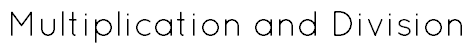MAFS.4.NBT.2.5: Multiply a whole number of up to four digits by a one-digit whole number, and multiply two two-digit numbers, using strategies based on place value and the properties of operations. Illustrate and explain the calculation by using equations, rectangular arrays, and/or area models. MAFS.4.NBT.2.6: Find whole-number quotients and remainders with up to four-digit dividends and one-digit divisors, using strategies based on place value, the properties of operations, and/or the relationship between multiplication and division. Illustrate and explain the calculation by using equations, rectangular arrays, and/or area models.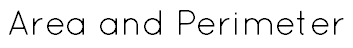MAFS.4.MD.2.4: Apply the area and perimeter formulas for rectangles in real world and mathematical problems. For example, find the width of a rectangular room given the area of the flooring and the length, by viewing the area formula as a multiplication equation with an unknown factor.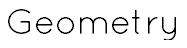MAFS.4.G.1.1: Draw points, lines, line segments, rays, angles (right, acute, obtuse), and perpendicular and parallel lines. Identify these in two-dimensional figures. MAFS.4.G.1.2: Classify two-dimensional figures based on the presence or absence of parallel or perpendicular lines, or the presence or absence of angles of a specified size. Recognize right triangles as a category, and identify right triangles. MAFS.4.G.1.3: Recognize a line of symmetry for a two-dimensional figure as a line across the figure such that the figure can be folded along the line into matching parts. Identify line-symmetric figures and draw lines of symmetry. MAFS.4.MD.3.5: Recognize angles as geometric shapes that are formed wherever two rays share a common endpoint, and understand concepts of angle measurement: An angle is measured with reference to a circle with its center at the common endpoint of the rays, by considering the fraction of the circular arc between the points where the two rays intersect the circle. An angle that turns through 1/360 of a circle is called a one-degree angle, and can be used to measure angles. An angle that turns through n one-degree angles is said to have an angle measure of n degrees. MAFS.4.MD.3.7: Measure angles in whole-number degrees using a protractor. Sketch angles of specified measure.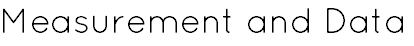MAFS.4.MD.1.1: Know relative sizes of measurement units within one system of units including km, m, cm; kg, g; lb, oz.; l, ml; hr, min, sec. Within a single system of measurement, express measurements in a larger unit in terms of a smaller unit. Record measurement equivalents in a two-column table. For example, know that 1 ft is 12 times as long as 1 in. Express the length of a 4 ft snake as 48 in. Generate a conversion table for feet and inches listing the number pairs (1, 12), (2, 24), (3, 36), ... MAFS.4.MD.1.2: Use the four operations to solve word problems involving distances, intervals of time, and money, including problems involving simple fractions or decimals. Represent fractional quantities of distance and intervals of time using linear models. MAFS.4.MD.2.4: Make a line plot to display a data set of measurements in fractions of a unit (1/2, 1/4, 1/8). Solve problems involving addition and subtraction of fractions by using information presented in line plots. For example, from a line plot find and interpret the difference in length between the longest and shortest specimens in an insect collection.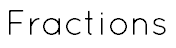MAFS.4.NF.1.1: Explain why a fraction a/b is equivalent to a fraction (n x a)/(n x b) by using visual fraction models, with attention to how the number and size of the parts differ even though the two fractions themselves are the same size. Use this principle to recognize and generate equivalent fractions. MAFS.4.NF.1.2: Compare two fractions with different numerators and different denominators, e.g., by creating common denominators or numerators, or by comparing to a benchmark fraction such as 1/2. Recognize that comparisons are valid only when the two fractions refer to the same whole. Record the results of comparisons with symbols >, =, or <, and justify the conclusions, e.g., by using a visual fraction model. MAFS.4.NF.2.3: Understand a fraction a/b with a > 1 as a sum of fractions 1/b. Understand addition and subtraction of fractions as joining and separating parts referring to the same whole. Decompose a fraction into a sum of fractions with the same denominator in more than one way, recording each decomposition by an equation. Justify decompositions, e.g., by using a visual fraction model. Examples: 3/8 = 1/8 + 1/8 + 1/8 ; 3/8 = 1/8 + 2/8 ; 2 1/8 = 1 + 1 + 1/8 = 8/8 + 8/8 + 1/8. Add and subtract mixed numbers with like denominators, e.g., by replacing each mixed number with an equivalent fraction, and/or by using properties of operations and the relationship between addition and subtraction. Solve word problems involving addition and subtraction of fractions referring to the same whole and having like denominators, e.g., by using visual fraction models and equations to represent the problem. MAFS.4.NF.2.4: Apply and extend previous understandings of multiplication to multiply a fraction by a whole number. Understand a fraction a/b as a multiple of 1/b. For example, use a visual fraction model to represent 5/4 as the product 5 x (1/4), recording the conclusion by the equation 5/4 = 5 x (1/4). Understand a multiple of a/b as a multiple of 1/b, and use this understanding to multiply a fraction by a whole number. For example, use a visual fraction model to express 3 x (2/5) as 6 x (1/5), recognizing this product as 6/5. (In general, n x (a/b) = (n x a)/b.) Solve word problems involving multiplication of a fraction by a whole number, e.g., by using visual fraction models and equations to represent the problem. For example, if each person at a party will eat 3/8 of a pound of roast beef, and there will be 5 people at the party, how many pounds of roast beef will be needed? Between what two whole numbers does your answer lie?